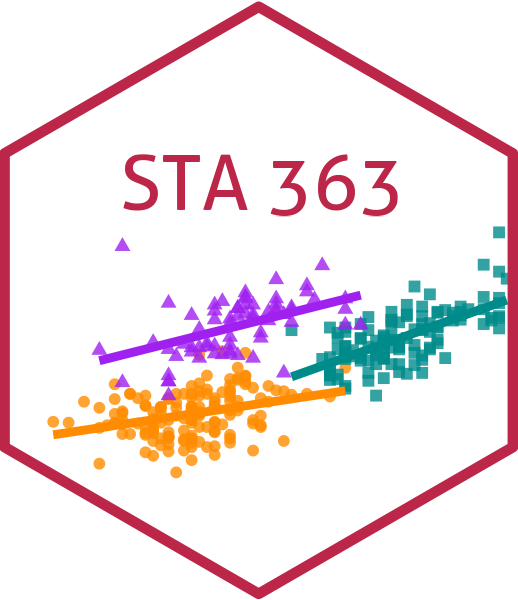# Plotting Decision trees

Lucy D’Agostino McGowan

## Plotting decision trees

There are several R packages that assist with tree plotting

• rpart.plot
• partykit
• rattle

## Where to find out more about packages

1. Vignettes
2. Journal Article (R Journal is great)
3. Rstudio Community
4. StackOverflow

## Learn about partykit

Google partykit to find out how more about it (hint: use key words like rstats and decision tree plots)

03:00

## rpart.plot

We’re going to focus on rpart.plot, but feel free to try the others!

install.packages("rpart.plot")
library(rpart.plot)

## rpart.plot

tree_spec <- decision_tree(
cost_complexity = 0.1,
tree_depth = 10,
mode = "regression") |>
set_engine("rpart")

wf <- workflow() |>
recipe(Salary ~ Hits + Years + PutOuts + RBI + Walks + Runs,
data = baseball)
) |>
model <- fit(wf, baseball)
rpart.plot(model$fit$fit$fit, roundint = FALSE) ## rpart.plot rpart.plot(model$fit$fit$fit,
roundint = FALSE)
## Application Exercise
2. Install rpart.plot
10:00